## 9.4.12 Mathematical Spacing

LaTeX deals with mathematical spacing fairly well, but sometimes you may find you want to adjust the spacing yourself. Available spacing commands are listed in Table 9.15.

 Example Input Example Output Command $AB$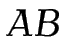\thinspace or \, $A\,B$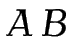\medspace† or \: $A\:B$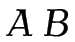\thickspace† or \; $A\;B$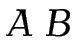\quad $A\quad B$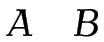\qquad $A\qquad B$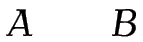\negthinspace or \! $A\!B$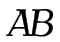\negmedspace† $A\negmedspace B$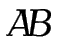\negthickspace† $A\negthickspace B$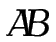Exercise 24: More Mathematics

This exercise uses the spacing command \qquad. In addition, it has a function name, diag, and it uses the \forall and ellipses symbols. It also redefines the \vec command, as was done in the previous section, uses the bmatrix environment (see §9.4.10. Arrays), and has subscripts and superscripts.

Try to reproduce the following output: